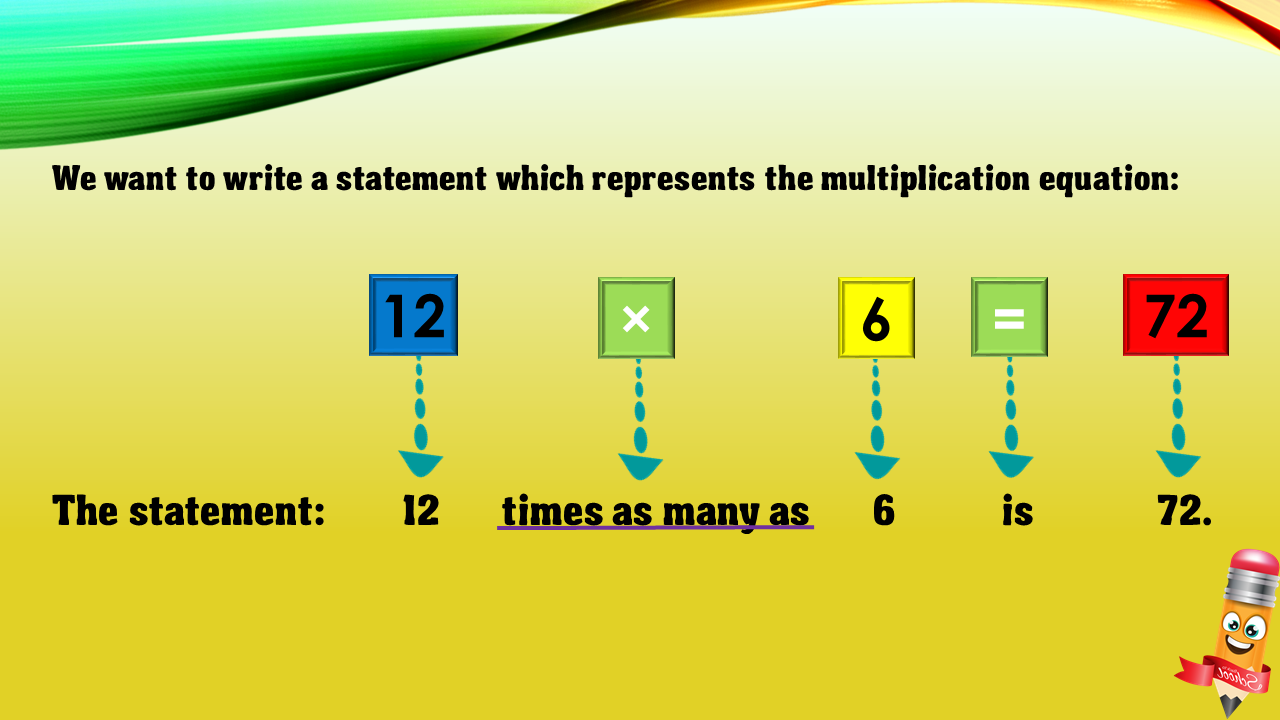1
visibility

Choose one statement which represents the equation:
12 × 6 = 72 ?

• A

12 more than 6 is 72.

• B

6 less than 72 is 12.

• C

12 times as many as 6 is 72.

• D

6 times as many as 72 is 12.